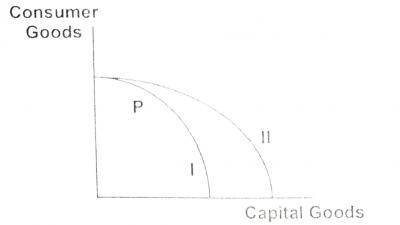# Economics 2018 Past Questions | JAMB

Study the following Economics past questions and answers for JAMBWAEC  NECO and Post-JAMB. Get prepared with official past questions and answers for upcoming examinations.

11.  The minimum number of share holders for joint stock companies is_______________

• A. 4
• B. 2
• C. 7
• D. 3

12.  If a demand curve that intersects a perfectly inelastic supply curve shift is rightward, then____________

• A. only the quantity will increase
• B. The prices will remain constant
• C. the equilibrium price and quantity will increase
• D. only the price will increase

13.  The basic economic problems of society include___________

• A. scarcity, for whom to produce and where
• B. what to produce, how and for whom
• B. scarcity, when to produce and how
• D. how to produce and sell

14.  In an open economy, the GNP is measured as_____________?

• A. C + 1 + G(X-M)
• B. C + 1 + G
• C. C + 1 + G + X
• D. C + 1 + G + M

15.  In a developing economy, productivity is measured by the____________

• A. output – labour ratio
• B. capital – output ratio
• C. output growth rate
• D. Output per capital

16.  The need to construct a scale of preference is necessitated by____________

• A. scarcity and the need for choice
• B. The needs to satisfy wants
• C. Non – availability of factors of production
• D. Scarcity of resources

17.  The privatization exercise in Nigeria is a move towards a____________

• A. command economy
• B. mixed economy
• C. subsistence economy
• D. market economy

18.  The mean is the best measure of central tendency because it__________

• A. can be calculated from incomplete data
• B. is a balancing point in an observation
• C. is a midpoint value in an array of data
• D. is not affected by extreme values in a data

19.

 No of Men 0 1 2 3 4 5 6 7 8 Total Product(unit) 200 440 540 600 640 660 660 640

If the price per unit  is N2, what is the average revenue when 6 men are employed?

• A. N110
• B. N120
• C. N220
• D. N330

20.The current stage of the economy is depicted in curve I. The movement of curve I to II suggests___________

• A. an Improvement in capital goods technology but not In consumer goods technology
• B. a movement from unemployment to full employment
• C. that the output for the society has declined
• D. a gain in consumer goods technique and not in capital goods technique

## All Subjects

Subscribe
Notify ofInline Feedbacks